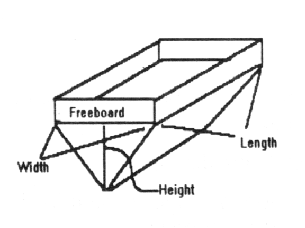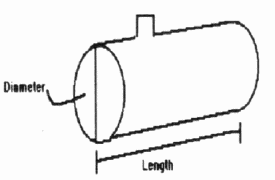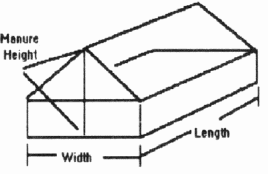##AY-278

### Soils Purdue University Cooperative Extension Service West Lafayette, IN 47907

#### Brad C. Joern and Sarah L. Brichford Department of Agronomy

Good manure management is an essential skill for today's livestock producer. Proper storage, handling, and land application of manure is necessary to utilize manure nutrients for crop production and avoid problems with environmental contamination. Developing a strategy for land application of manure begins with knowing the amount of manure contained in a spreader load. With this information and a laboratory analysis of your livestock manure, you can calculate the application rate of both the manure and the manure nutrients (N, P, and K) on a per acre basis. This publication describes how to estimate the capacity of three types of manure spreaders: liquid tank, V-bottom, and box. A separate publication, AY- 277, contains instructions for obtaining a manure sample and worksheets for calculating manure application rates after you have estimated manure spreader capacity.

Estimating manure spreader capacity is a simple procedure. Your owner's manual should provide information regarding the size or capacity of the spreader. If this information is not available, use the following formulas to calculate capacity. Simply make the necessary measurements for your spreader type and use these values in the formula given.

### V-bottom TypeV-bottom spreaders can hold either semi-solid (gallons) or solid manure (tons). If you do not have the capacity of your spreader, you will have to make three measurements.

1. Measure the length and width (in feet) of the manure spreader box. Then, measure the height from the bottom of the spreader box to the top of the manure load.

2. Insert these values into the following formula:

Length (ft.) x Width (ft.) x Height (ft.) x 0.016 = Capacity in tons

Length (ft.) x Width (ft.) x Height (ft.) x 3.75= Capacity in gallons

Solid _______ (ft.) x ______ (ft.) x _____ (ft.) x 0.016 = _______ tons

Liquid _______ (ft.) x ______ (ft.) x _____ (ft.) x 3.75 = _______ gallons

### Liquid Tank TypeThe capacity of a liquid tank spreader is the easiest to calculate. The capacity is often painted on the side of the tank. If capacity information is not available, use the following steps to calculate the capacity (in gallons) of your spreader.

1.Measure the length and diameter of the tank (in feet) as shown in the diagram.

2.Insert these values into the following formula:

[Diameter (ft.) / 2] 2 x Length (ft.) x 23.6 = Capacity in gallons

Your spreader: [ ft / 2]2 x ______ ft. x 23.6 = _______ gallons

### Box TypeThe capacity of a box spreader is difficult to estimate accurately because the density of solid manure is quite variable. Density is the weight of the manure per volume of manure (e.g., lb./ft. 3). Manure density varies depending on the amount of bedding used. Therefore, if you estimate spreader capacity as only the volume of manure the spreader holds you are overlooking the fact that some manure weighs more than other manure. This can cause a significant error when calculating manure application rates.

To account for the variability in manure density, we recommend weighing five, full spreader loads and then calculating the average weight of a full spreader. This is the preferred method and will give you a reasonable estimate of spreader capacity.

1. Weigh five different spreader loads, using drive-on scales or portable truck scales,* and calculate the average weight. Remember, one ton equals 2000 pounds.

Total Weight _______ / 5 = Average Loaded Weight _______ tons

2. Weigh the spreader when it is empty, and subtract this value from the average loaded weight. The resulting value is the manure holding capacity.

Average Loaded Weight _______ - Empty Spreader Weight ________ = ________ tons

*If you do not have access to scales you can calculate spreader volume by measuring three dimensions of the spreader. Keep in mind that this method is much less accurate than weighing.

1. Measure the length and width of the manure spreader box (in feet) as shown in the diagram.

2. Fill the spreader and measure the distance from the bottom of the box to the top of the manure load. This is the manure height. Take five measurements and use the average value in the formula.

3.Insert these values into the following formula:

Length (ft.) x Width (ft.) x Manure Height (ft.) x 0.025 = Capacity in tons

Your spreader: ______ ft. x _____ ft. x _____ ft. x 0.025 = _______ tons

New 9/93

Editor: Cheri Janssen, Department of Agronomy

Cooperative Extension work in Agriculture and Home Economics, state of Indiana, Purdue University, and U.S. Department of Agriculture cooperating; H. A. Wadsworth, Director, West Lafayette, IN. Issued in furtherance of the acts of May 8 and June 30, 1914. The Cooperative Extension Service of Purdue University is an affirmative action/equal opportunity institution.